# 在MATLAB中调用 Python

``````py.list({'This','is a','list'})      % Calls built-in function list
py.textwrap.wrap('This is a string') % Calls wrap function in module textwrap
``````

MATLAB 中的 Python 库 – MATLAB & Simulink – MathWorks 中国

### 检查环境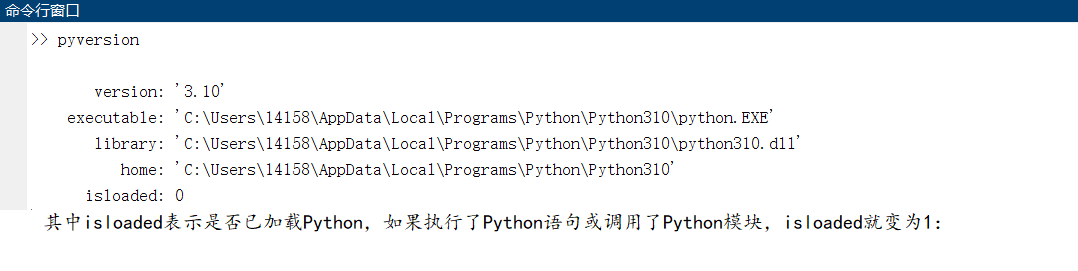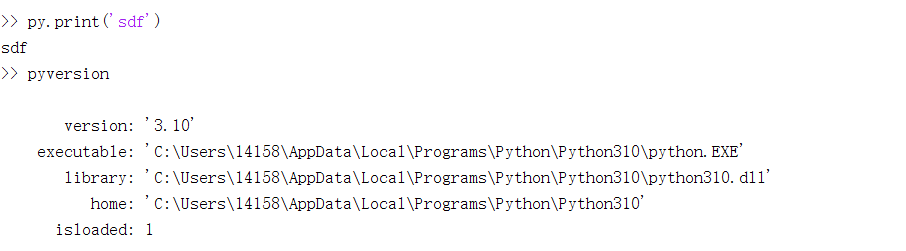`pyenv` 也可以检测验证是否安装了受支持的版本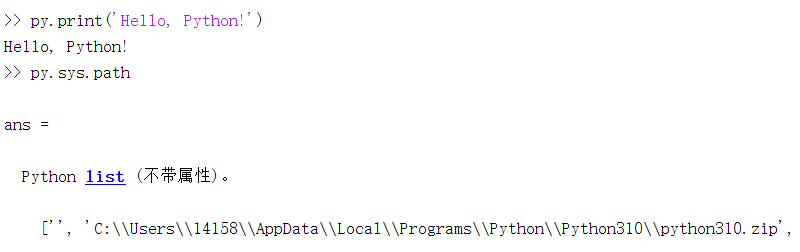### 函数调用

``````# myfun.py
def test():
print('Hello, Matlab!')

c = a + b
return c
``````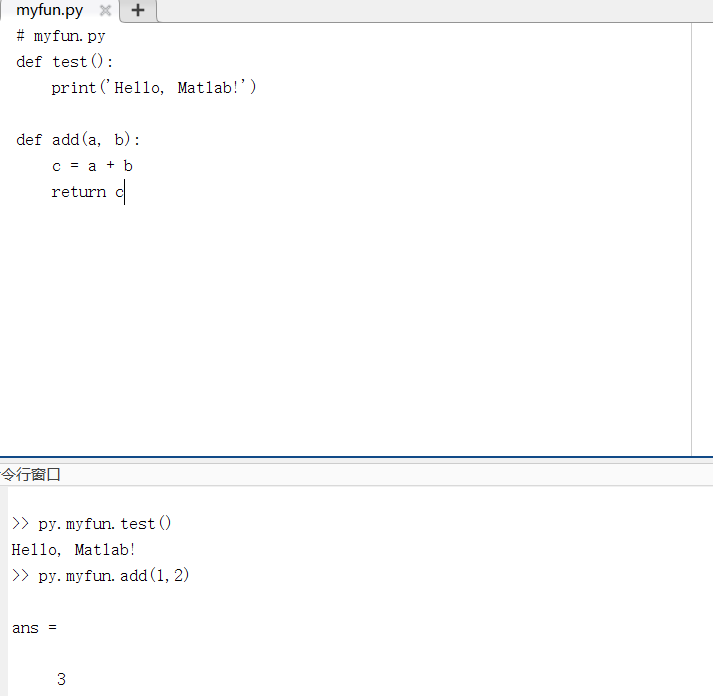``````% 重载Python模块
clear classes
obj = py.importlib.import_module('myfun');
``````

### 类调用

``````class myclass():
def __init__(self):
self.count = 0
self.count = self.count + 1
print('count = ', self.count)
``````

``````mycalss = py.myfun.myclass;
for i = 1:5
end
``````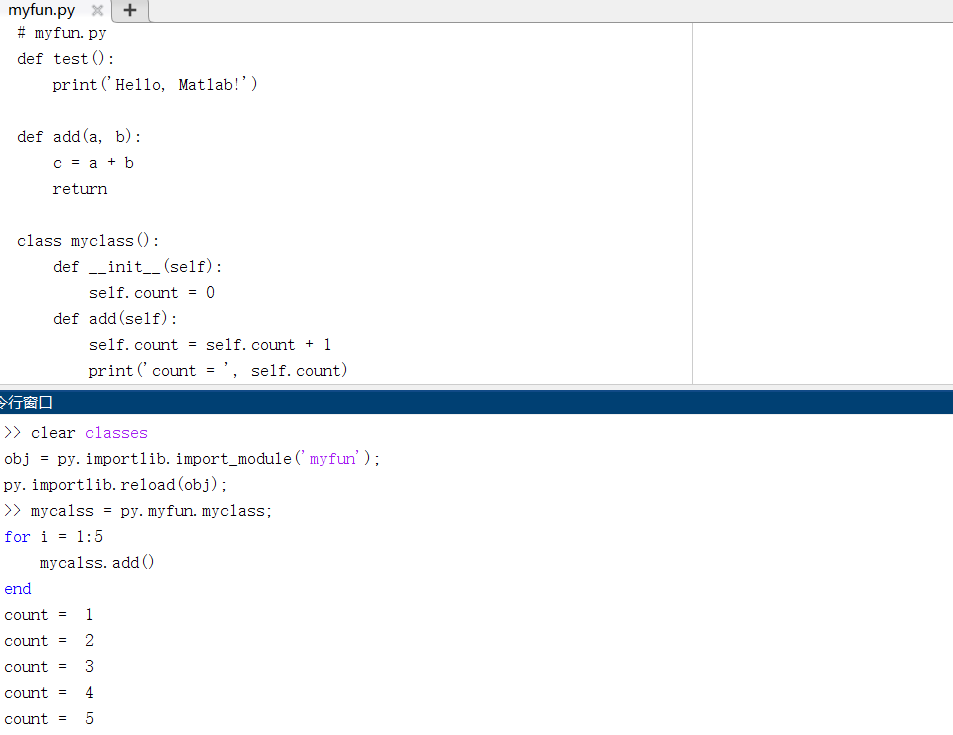### Python 帮助文档

``````py.help('list.append')
Help on method_descriptor in list:

list.append = append(...)
L.append(object) -> None -- append object to end
``````

### 创建数据

Python `list``[]` MATLAB `py.list`
`>>> ['Robert', 'Mary', 'Joseph']` `>> py.list({'Robert','Mary','Joseph'})`
`>>> [[1,2],[3,4]]` `>> py.list({py.list([1,2]),py.list([3,4])})`
Python `tuple``()` MATLAB `py.tuple`
`>>> ('Robert', 19, 'Biology')` `>> py.tuple({'Robert',19,'Biology'})`
Python `dict``{}` MATLAB `py.dict`
`>>> {'Robert': 357, 'Joe': 391, 'Mary': 229}` `>> py.dict(pyargs(...'Robert',357,'Mary',229,'Joe',391))` 有关传递关键字参数的信息，请参阅 `pyargs`